# Scientific Notation Review Scientific notation expresses a number

• Slides: 12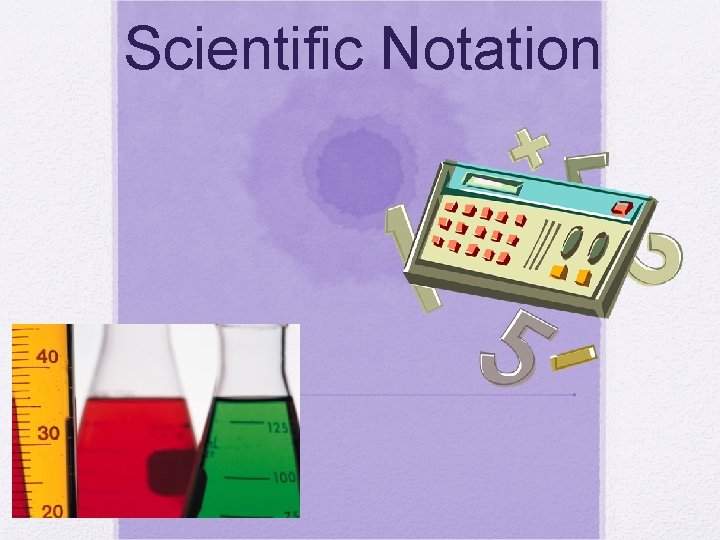Scientific NotationReview: Scientific notation expresses a number in the form: M x Any number between 1 and 10 n is an integer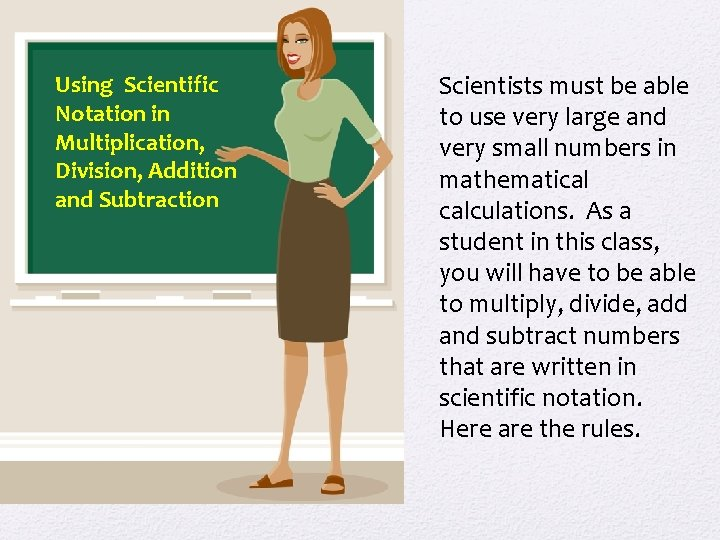Using Scientific Notation in Multiplication, Division, Addition and Subtraction Scientists must be able to use very large and very small numbers in mathematical calculations. As a student in this class, you will have to be able to multiply, divide, add and subtract numbers that are written in scientific notation. Here are the rules.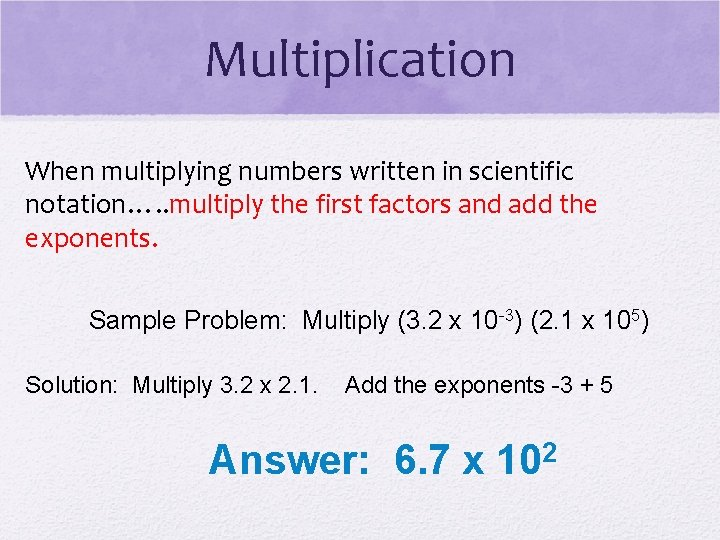Multiplication When multiplying numbers written in scientific notation…. . multiply the first factors and add the exponents. Sample Problem: Multiply (3. 2 x 10 -3) (2. 1 x 105) Solution: Multiply 3. 2 x 2. 1. Add the exponents -3 + 5 Answer: 6. 7 x 102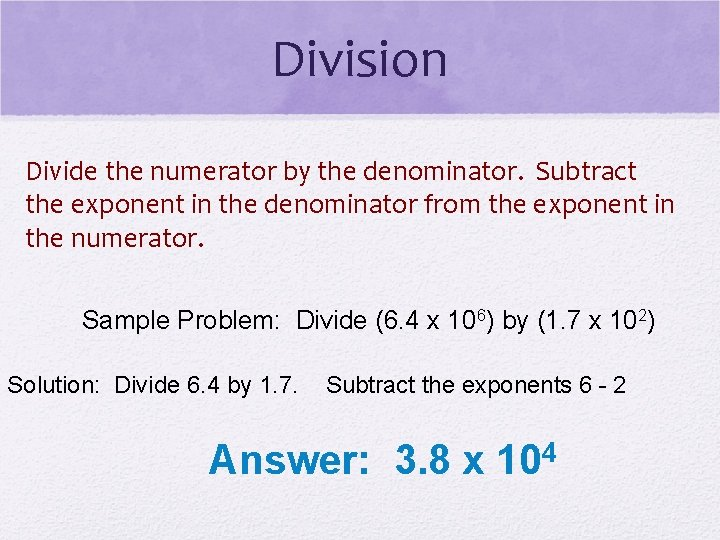Division Divide the numerator by the denominator. Subtract the exponent in the denominator from the exponent in the numerator. Sample Problem: Divide (6. 4 x 106) by (1. 7 x 102) Solution: Divide 6. 4 by 1. 7. Subtract the exponents 6 - 2 Answer: 3. 8 x 104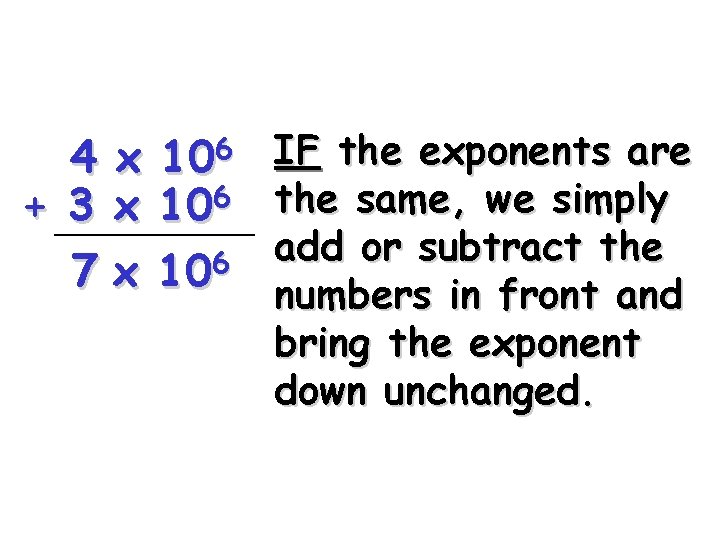6 10 4 x 6 + ________ 3 x 10 7 x 106 IF the exponents are the same, we simply add or subtract the numbers in front and bring the exponent down unchanged.4 + 3 106 x x 105 If the exponents are NOT the same, we must move a decimal to make them the same.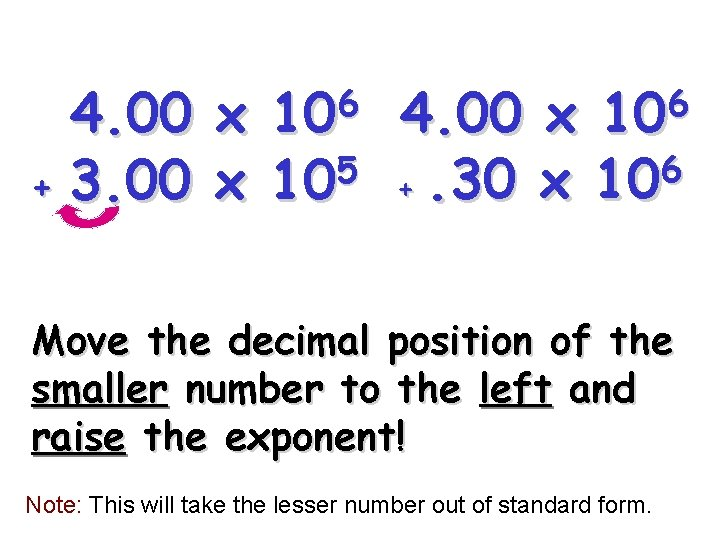6 10 4. 00 x 6 5 +. 30 x 10 + 3. 00 x 10 Move the decimal position of the smaller number to the left and raise the exponent! Note: This will take the lesser number out of standard form.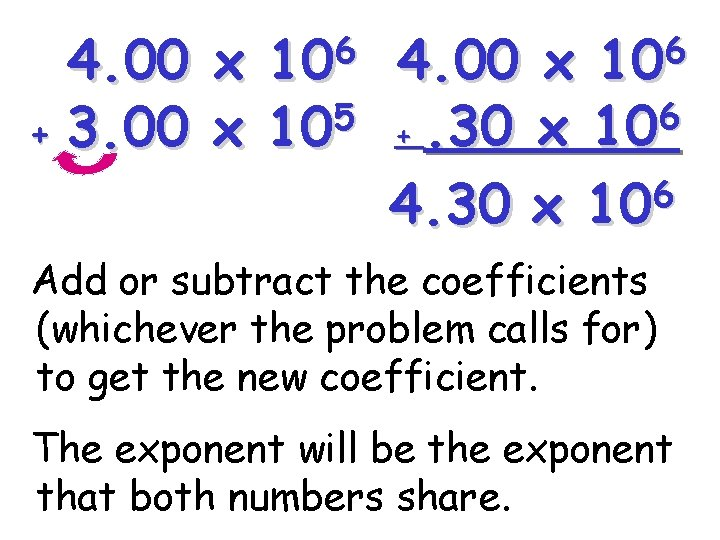4. 00 + 3. 00 6 10 x 4. 00 x 6 5 x 10 +. 30 x 10 6 4. 30 x 10 Add or subtract the coefficients (whichever the problem calls for) to get the new coefficient. The exponent will be the exponent that both numbers share.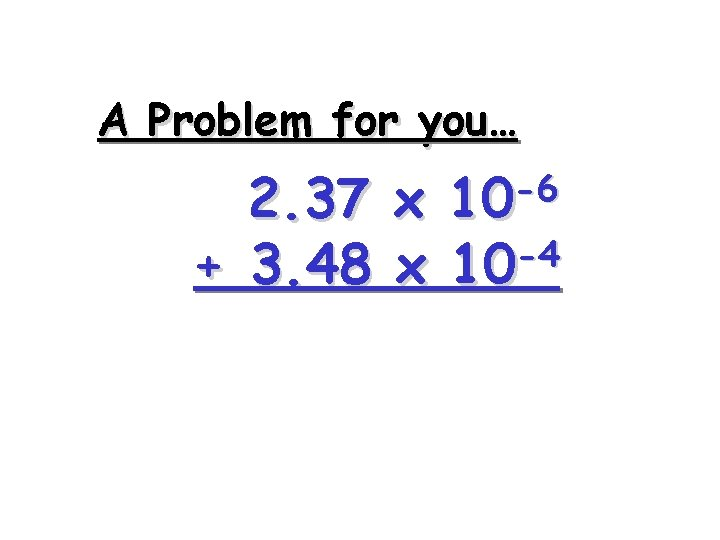A Problem for you… -6 10 2. 37 x -4 + 3. 48 x 10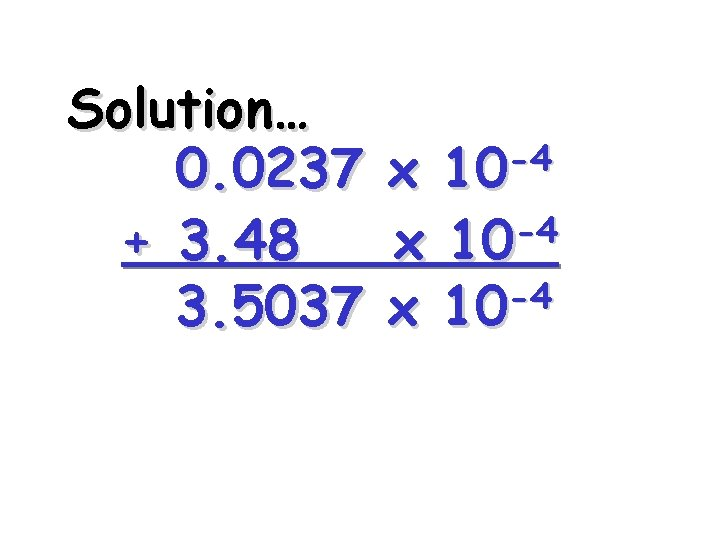Solution… 0. 0237 + 3. 48 3. 5037 -4 10 x -4 x 10Addition and Subtraction To add or subtract numbers written in scientific notation, you must…. express them with the same power of ten. Sample Problem: Add (5. 8 x 103) and (2. 16 x 104) Solution: Since the two numbers are not expressed as the same power of ten, one of the numbers will have to be rewritten in the same power of ten as the other. 5. 8 x 103 =. 58 x 104 so. 58 x 104 + 2. 16 x 104 =? Answer: 2. 74 x 104